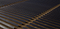# Python Data Structures: Your Starter Kit to Learning AlgorithmsThis is just meant as a friendly introduction to a topic that every computer science and data science program I know off explores in an entire course or a few.

Working with any kind of algorithm starts with learning a set of data structures associated with it. This makes sense since most algorithms work on some kind of data that must be stored and held somehow, somewhere. That’s where data structures come handy!

Data Structures are used to organize information and data in a variety of ways such that an algorithm can be applied to the structure in the most efficient way possible.

In this post, I will give a basic introduction to the most common data structures used. I will do so using the Python language. By the end of the post, you will gain your first introduction to arrays, linked lists, stacks, queues, and hash tables in Python.

Let’s get started!

# Arrays

An array is a collection of elements where the position of each element is defined by an index or a key value. A one-dimensional array, for example, contains a linear set of values. In an array, element position can be calculated mathematically thus enabling array elements to be accessed directly. Since the position of each element in the array can be directly computed, we do not need to navigate the entire data structure to be able to access a particular element.In Python, arrays are indexed starting at zero. In other words, the first element of the array is at index 0, the second at index 1, the third at 2 and so on. To access an element inside a one-dimensional array, we provide one index. For two-dimensional arrays, two indices are needed.

Time complexity of basic operations on arrays:

1. Calculating the index of an element in an array is a constant time operation. In Big-O notation, this is indicated by O(1). The operation is not dependent on how many elements the array contains.
2. Appending or deleting an element at the beginning or in the middle of an array is an operation with linear time complexity. In Big-O notation, this is indicated by O(n). This is because the remaining elements already present in the array will need to be moved to new locations in memory.
3. Appending or deleting an element at the end of an array is an operation of constant time complexity O(1). This is independent of the size of the array since we do not need to move other elements in the array to new locations in memory. We simply are adding an element to the end of the array.

Much like arrays, linked lists are made up of a linear collection of elements called nodes. They differ, however, in that each node contains a reference that identifies the next node in the list. The first node in a linked list is called the head. Each node contains a field that points to the next element in the list. The last node contains a field that points to null.Photo by Chris Leipelt on Unsplash

A_ singly-linked list_ is one where only one direction is provided at each node. In other words, each item in the list only has reference to its next neighboring node. A doubly-linked list is a list where each node has a reference to the neighboring node that precedes it and the one that follows it.

Unlike arrays, linked lists are a lot easier to deal with as far as inserting and removing elements is concerned. Elements inside a linked list do not need to be rearranged entirely to add or remove an element, thus not requiring any memory reorganization. This is simply because we can manipulate the pointers to nodes instead of reorganizing the nodes themselves. The downside to linked lists, however, is that you can not do random item access in constant time.

## Learn Programming, Software Engineering, Machine Learning, And More

Best Free Resources to Learn Programming, Software Engineering, Machine Learning, And More All you need to learn. Do you know that you can take the courses from MIT, Stanford.

## Here’s how I Learned Just Enough Programming for Data Science

How to approach learning programming and best books I recommend. There’s no doubt that data science requires decent programming skills, but how much is enough?

## “How’d you get started with machine learning and data science?”

“How’d you get started with machine learning and data science?”: I trained my first model in 2017 on my friend's lounge room floor.

## Learn Data Science Today - Data Science Tutorial for Beginners 2020!

How and why to start Learning to be a data scientist in 2020! This Data Science Course will give you a Step by Step idea about the Data Science Career, Data science Hands-On Projects, roles & salary offered to a Data Scientist!

## Data Science Course in Dallas

Become a data analysis expert using the R programming language in this [data science](https://360digitmg.com/usa/data-science-using-python-and-r-programming-in-dallas "data science") certification training in Dallas, TX. You will master data...